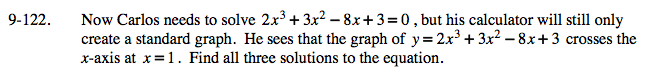### Home > A2C > Chapter 9 > Lesson 9.3.1 > Problem9-122

9-122.Divide 2x3 + 3x2 − 8x + 3 = 0 by x − 1.

$\frac{2\textit{x}^{3}+3\textit{x}^{2}-8\textit{x}+3}{\textit{x}-1}=2\textit{x}^{2}+5\textit{x}-3$

Now factor the equation.

2x2 + 3x2 − 8x + 3 = 0
(x − 1)(2x2 + 5x − 3) = 0

Continue to factor and find the three solutions.

$x=1, \frac{1}{2}, -3$